# Python实战技巧：快速构建神经网络

## 1.前言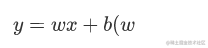和是常数） 我们给定一个x，就能得到一个y。只不过神经网络的函数要比上面的函数复杂得多。

## 2.机器学习

### 2.1、什么是机器学习？

``````1, 3, 5, 7, 9

## 3.线性回归和逻辑回归

### 3.1、线性回归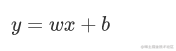``````1, 3, 5, 7, 9

``````x, y
1, 1
2, 3
3, 5,
4, 7,
5, 9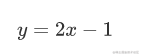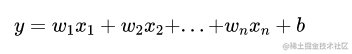### 3.2、线性回归实战

``````pip install scikit-learn

``````import numpy as np
from sklearn.linear_model import LinearRegression
# 准备x的数据
X = np.array([
,
,
,
,

])
# 准备y的数据
y = np.array([1, 3, 5, 7, 9])
# 创建线性回归模块
lr = LinearRegression()
# 填充数据并训练
lr.fit(X, y)
# 输出参数
print("w=", lr.coef_, "b=", lr.intercept_)

``````w= [2.] b= -1.0

``````import numpy as np
from sklearn.linear_model import LinearRegression
X = np.array([    ,
,
,
,

])
y = np.array([1, 3, 5, 7, 9])
lr = LinearRegression()
lr.fit(X, y)
y_predict = lr.predict(np.array([]))
print(y_predict)

### 3.3、逻辑回归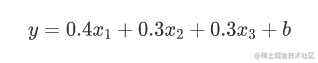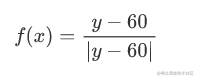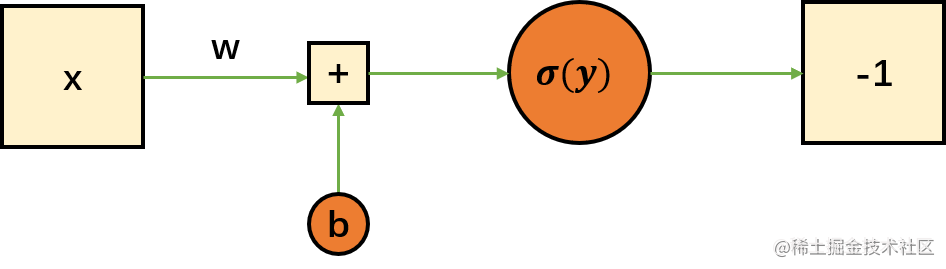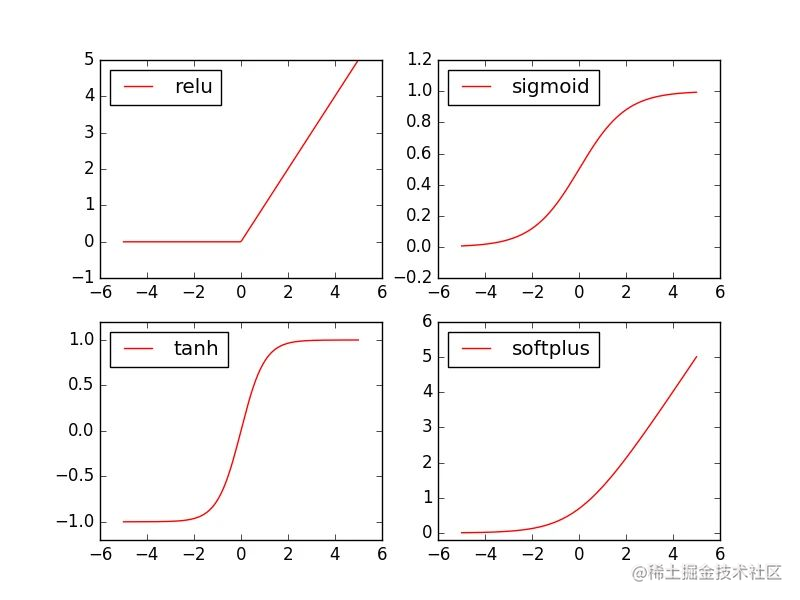### 3.4、逻辑回归实战

``````import numpy as np
from sklearn.linear_model import LogisticRegression
# 准备X的数据
X = np.array([
,
,
,
,
,

])
# 准备y的数据
y = np.array([1, 0, 0, 1, 0, 1])
# 创建逻辑回归模型
lr = LogisticRegression()
# 填充数据并训练
lr.fit(X, y)
# 准备用于测试的数据
X_test = np.array([
,
,
,

])
# 判断测试数据是否及格
y_predict = lr.predict(X_test)
print(y_predict)

``````[1 1 0 0]

## 4. 神经网络

### 4.1、神经网络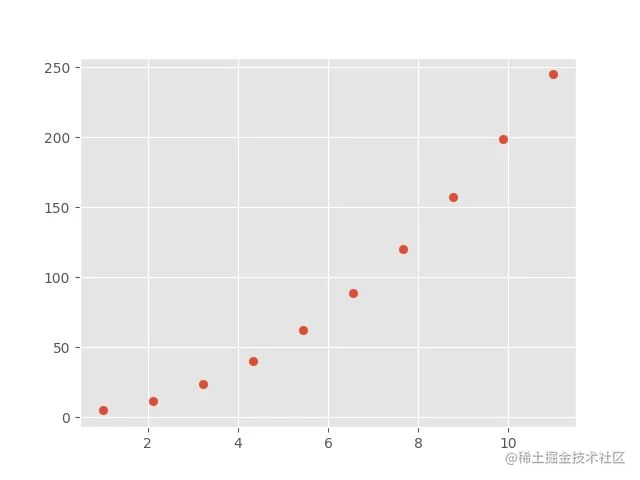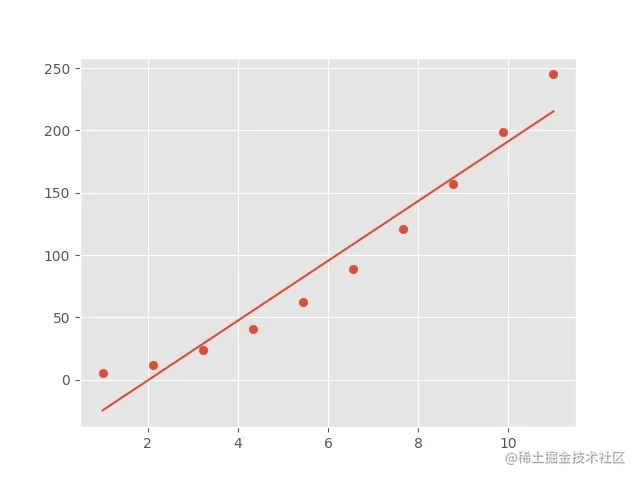### 4.2、输入层、隐层、输出层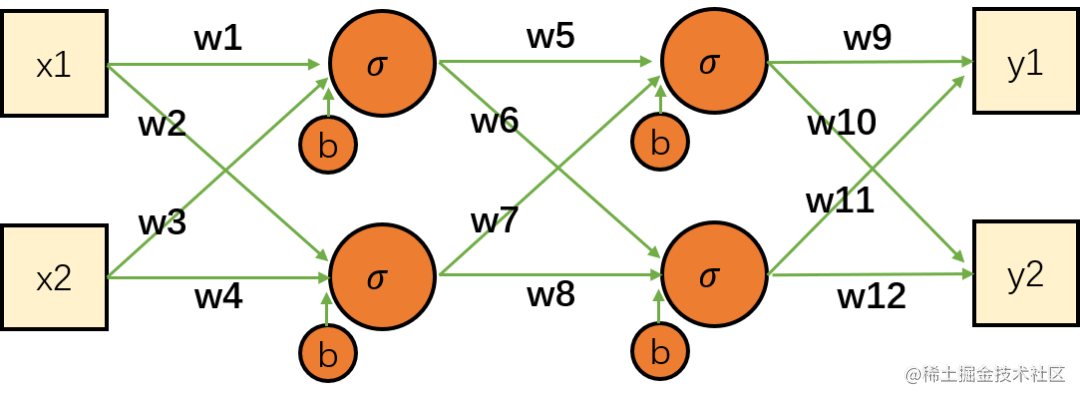### 4.3、神经网络实战

``````from sklearn.datasets import load_iris
from sklearn.neural_network import MLPClassifier
from sklearn.model_selection import train_test_split

# 加载数据集
# 拆分数据集
X_train, X_test, y_train, y_test = train_test_split(iris_data['data'], iris_data['target'], test_size=0.25, random_state=1)
# 创建神经网络模型
mlp = MLPClassifier(solver='lbfgs', hidden_layer_sizes=[4, 2], random_state=0)
# 填充数据并训练
mlp.fit(X_train, y_train)
# 评估模型
score = mlp.score(X_test, y_test)
print(score)

``````0.9210526315789473